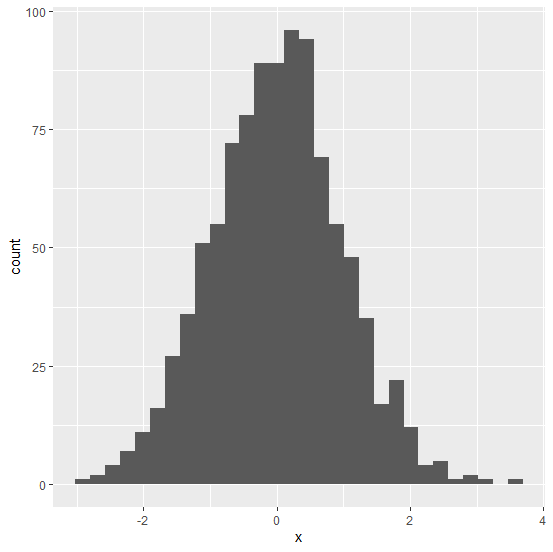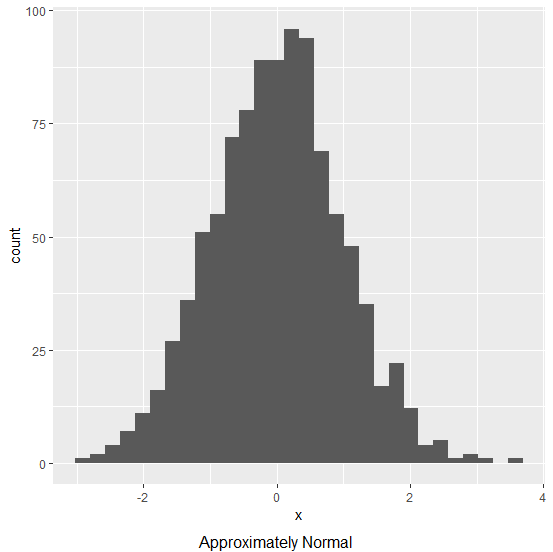# How to set the chart title at the bottom using ggplot2 in R?

Generally, the chart title is written on the upper side of the plot but sometimes we need to put it in the bottom. This is recommended in situations when the chart title explains something about the plot. For example, if we are plotting a normal distribution then we can use “Approximately Normal” as the chart title in the bottom because we know that perfect normal is a very rare event. If we want to set the chart title at the bottom in a chart created by using ggplot2 then we need to use grid.arrange function of gridExtra package.

## Example

Consider the below data frame −

x<−rnorm(1000)
df<−data.frame(x)

library(ggplot2)
ggplot(df,aes(x))+geom_histogram(bins=30)

## OutputAdding the chart title at the bottom of the chart −

library(gridExtra)
grid.arrange(ggplot(df,aes(x))+geom_histogram(bins=30),bottom="Approximately Normal")

## OutputUpdated on: 07-Nov-2020

861 Views